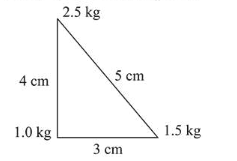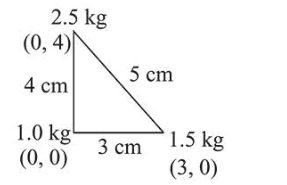# Three point particles of massesQuestion:

Three point particles of masses $1.0 \mathrm{~kg}, 1.5 \mathrm{~kg}$ and $2.5 \mathrm{~kg}$ are placed at three corners of a right angle triangle of sides $4.0 \mathrm{~cm}, 3.0 \mathrm{~cm}$ and $5.0 \mathrm{~cm}$ as shown in the figure. The center of mass of the system is at a point:1. (1) $0.6 \mathrm{~cm}$ right and $2.0 \mathrm{~cm}$ above $1 \mathrm{~kg}$ mass

2. (2) $1.5 \mathrm{~cm}$ right and $1.2 \mathrm{~cm}$ above $1 \mathrm{~kg}$ mass

3. (3) $2.0 \mathrm{~cm}$ right and $0.9 \mathrm{~cm}$ above $1 \mathrm{~kg}$ mass

4. (4) $0.9 \mathrm{~cm}$ right and $2.0 \mathrm{~cm}$ above $1 \mathrm{~kg}$ mass

Correct Option: 4,

Solution:

(4)$X_{\mathrm{cm}}=\frac{m_{1} x_{1}+m_{2} x_{2}+m_{3} x_{3}}{m_{1}+m_{2}+m_{3}}$

$X_{c m}=\frac{1 \times 0+1.5 \times 3+2.5 \times 0}{1+1.5+2.5}=\frac{1.5 \times 3}{5}=0.9 \mathrm{~cm}$

$Y_{\mathrm{cm}}=\frac{m_{1} y_{1}+m_{2} y_{2}+m_{3} y_{3}}{m_{1}+m_{2}+m_{3}}$

$Y_{c m}=\frac{1 \times 0+1.5 \times 0+2.5 \times 4}{1+1.5+2.5}=\frac{2.5 \times 4}{5}=2 \mathrm{~cm}$

Hence, centre of mass of system is at point $(0.9,2)$